NEET and AIPMT NEET Physics Magnetism and Matter MCQ Questions Solved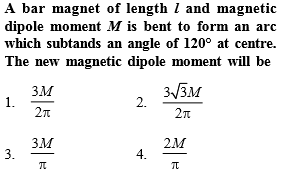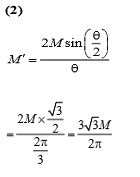Difficulty Level:

• 24%
• 46%
• 17%
• 15%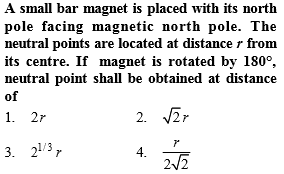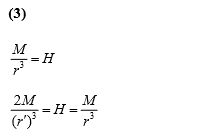Difficulty Level:

• 20%
• 31%
• 32%
• 19%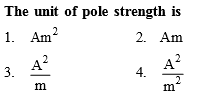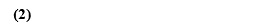Difficulty Level:

• 30%
• 56%
• 11%
• 5%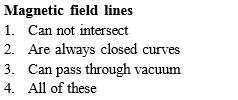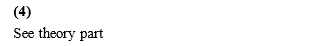Difficulty Level:

• 13%
• 6%
• 4%
• 78%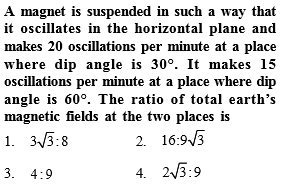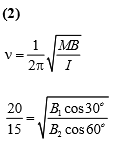Difficulty Level:

• 14%
• 57%
• 22%
• 9%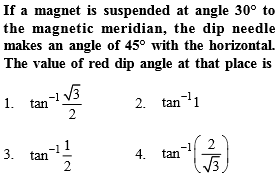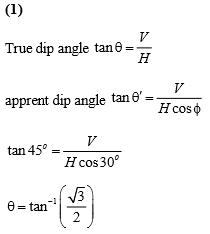Difficulty Level:

• 27%
• 23%
• 15%
• 37%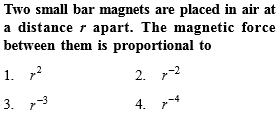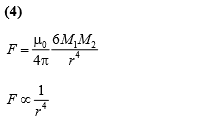Difficulty Level:

• 19%
• 36%
• 25%
• 22%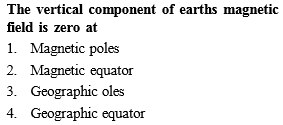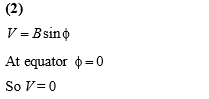Difficulty Level:

• 28%
• 45%
• 9%
• 20%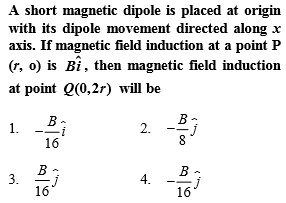(1)

$\mathrm{B}=\frac{{\mathrm{\mu }}_{0}}{4\mathrm{\pi }}\frac{2\mathrm{M}}{{\mathrm{r}}^{3}}\phantom{\rule{0ex}{0ex}}\mathrm{B}\text{'}=\frac{{\mathrm{\mu }}_{0}}{4\mathrm{\pi }}\frac{\mathrm{M}}{8{\mathrm{r}}^{3}}\phantom{\rule{0ex}{0ex}}\mathrm{B}\text{'}=\frac{\mathrm{B}}{16}$

Difficulty Level:

• 21%
• 32%
• 23%
• 26%

The magnetic field at a point x on the axis of a small bar magnet is equal to the field at a point y on the equator of the same magnet. The ratio of the distances of x and y from the centre of the magnet is
(a) ${2}^{-3}$

(b) ${2}^{-1}{3}}$

(c) ${2}^{3}$

(d) ${2}^{1}{3}}$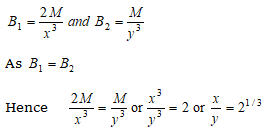Difficulty Level:

• 7%
• 38%
• 10%
• 47%